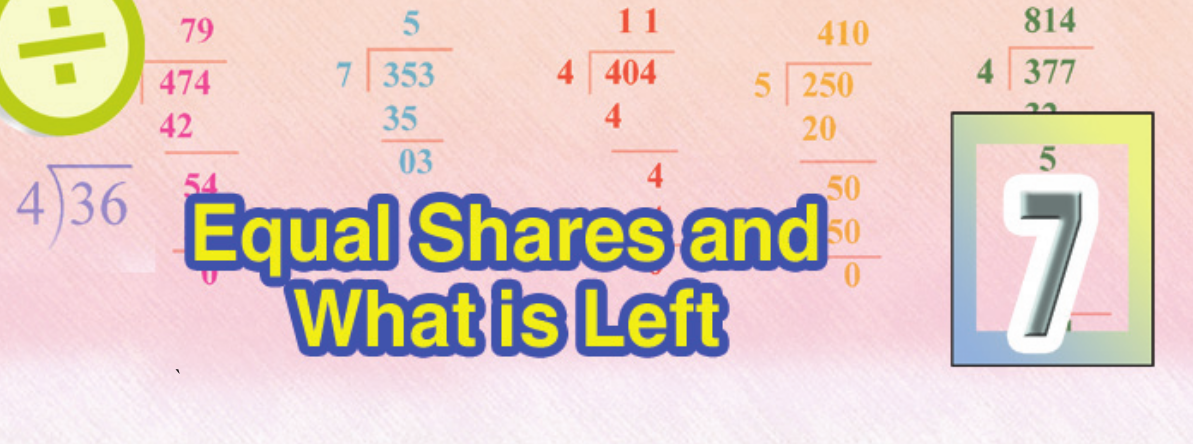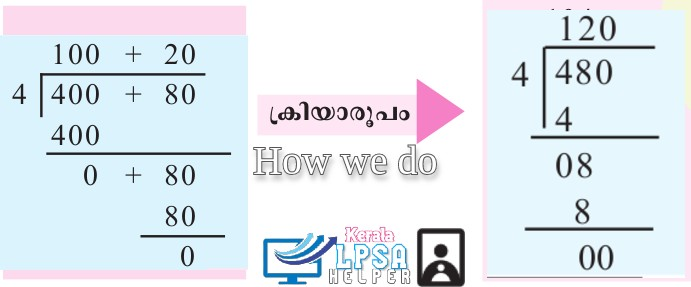## RELATED POSTSAn LP School uses 6 kilograms of mung for lunch. 135 kilograms of mung were bought. For how many days will it last?
Here we must divide 135 by 6.
Try it?
If is enough for 22 days. .
How much will be left after that?
3 KilogramsWhat remains after division is, called remainder.
How do we split 624 into 6 equal parts?
624 = 600 + 20 + 4
If 600 is divided into 6 equal parts, how much will be there in each
part?
That is 600 ÷ 6 = 100
How much 10 left? 24
24 divided into 6 is 24 ÷ 6 = 4
So, 624 ÷ 6 = (600 ÷ 6) + (24 ÷ 6) = 104
Multiplying the quotient by the divisor and adding the remainder gives the dividend. .
480 is to be divided by 4
For that, we need to divide 400 by 4 and 80 by 4 and add
400 divided by 4 is 100
80 divided by 4 is 20
480 divided by 4 is 120Find
Raju had 140 gooseberries in his hand. He sold 5 gooseberries for Rs 8. How much he earned from 140 gooseberries?